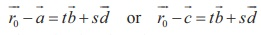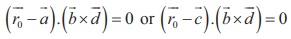Home | | Maths 12th Std | Equation of plane containing two non-parallel coplanar lines

# Equation of plane containing two non-parallel coplanar lines

(a) Parametric form of vector equation (b) Non-parametric form of vector equation (C) Cartesian form of equation of plane

Equation of plane containing two non-parallel coplanar lines

## (a) Parametric form of vector equation

Letbe two non-parallel coplanar lines. Then×≠. Let P be any point on the plane and let0 be its position vector. Then, the vectorsare also coplanar. So, we get. Hence, the vector equation in parametric form is.

## (b) Non-parametric form of vector equation

Letbe two non-parallel coplanar lines. Then×≠. Let P be any point on the plane and let0 be its position vector. Then, the vectorsare also coplanar. So, we get. Hence, the vector equation in non-parametric form is.

## (C) Cartesian form of equation of plane

In Cartesian form the equation of the plane containing the two given coplanar lines### Example 6.46

Show that the linesare coplanar. Also,find the non-parametric form of vector equation of the plane containing these lines.

### Solution

Comparing the two given lines withWe know that the two given lines are coplanar ,Therefore the two given lines are coplanar.Then we find the non parametric form of vector equation of the plane containing the two given coplanar lines. We know that the plane containing the two given coplanar lines iswhich implies that  (- (-iˆ - 3ˆj - 5kˆ)).(7iˆ -14ˆj + 7kˆ) = 0 . Thus, the required non-parametric vector equation of the plane containing the two given coplanar lines is. (iˆ - 2ˆj + ˆk ) = 0.

Study Material, Lecturing Notes, Assignment, Reference, Wiki description explanation, brief detail
12th Mathematics : UNIT 6 : Applications of Vector Algebra : Equation of plane containing two non-parallel coplanar lines |